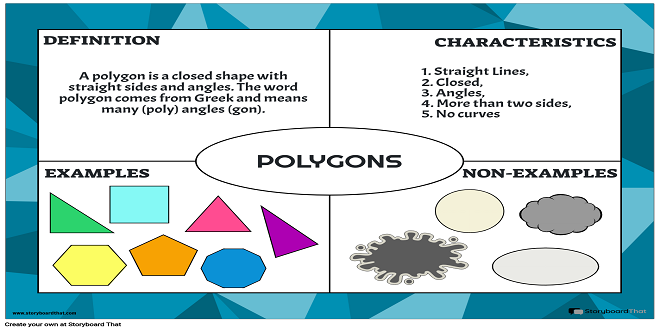# What is Polygon Shape?

In mathematics, shapes are considered an important topic because they help you identify diverse kinds of objects in your day-to-day life. Shapes of objects can vary depending on a number of features like a number of sides, length of sides, area, volume, angle, and much more. One shape that we come across very frequently is the polygon shape. A polygon is a closed figure that has a definable number of sides. It is a shape that has dimensions of height and width and has to have at least more than three sides.

This article will discuss polygons and their types with examples in detail.

## What is a Polygon?

Polygon is a Greek word where “poly” means many and “gon” means angle. In other words, a polygon is a closed shape that has at least three sides, three vertices and three angles.

Before we understand the types of polygons in detail let us understand three terms that are important when it comes to polygons.

First, edges. Edges in simple words refer to the sides of a polygon. For example, a triangle has three sides which means a triangle has three edges.

Second, vertices. Vertices are the points where two edges meet. They are referred to as points where the sides of the polygon join each other.

Third, diagonals. Diagonals refer to straight lines that connect two vertices. One thing to note is that diagonals only connect two vertices that are opposite to each other.

Now that we have understood the basic terms related to polygons, let’s dive deep to understand the classification of polygons.

## Classification of Polygons on the Basis of Angles & Sides

Polygons are classified on the basis of the relationship between angles and sides. The types of polygons under this category are:

• Regular Polygon: A regular polygon is a polygon that has equal interior angles. Additionally, a regular polygon also has equal sides.
• Irregular Polygon: An irregular polygon is a polygon in which the interior angles are not equal. Additionally, it also does not have equal sides.
• Convex Polygon: A convex polygon is one in which the inner angles are lesser than 180 degrees and the vertices are always pointing outwards.
• Concave Polygon: A concave polygon is a polygon in which one of the angles is more than 180 degrees.
• Quadrilateral Polygon: Polygons with four sides, four angles and four vertices are called quadrilateral polygons.
• Pentagon Polygons: Polygons that have five sides, five angles and five vertices are called pentagon polygons.

## Classification of Polygons on the Basis of Sides

Polygons are classified on the basis of the sides. The types of polygons under this category are:

• Triangle: A polygon with three sides, three angles and three vertices is called a triangle.
• Quadrilateral: A polygon with four sides, four angles, and four vertices is called a quadrilateral.
• Pentagon: A polygon with five sides, five angles, and five vertices is called a pentagon.
• Hexagon: A polygon with six sides, six angles, and six vertices is called a hexagon.
• Heptagon: A polygon with seven sides, seven angles, and seven vertices is called a heptagon.
• Octagon: A polygon with eight sides, eight angles, and eight vertices is called an octagon.
• Nonagon: A polygon with nine sides, nine angles, and nine vertices is called a nonagon.
• Decagon: A polygon with ten sides, ten angles, and ten vertices is called a decagon.

## Cuemath

Math might be tedious at times, but Cuemath is here to help. Cuemath was founded in India a few years ago with the goal of improving the way kids learn math. They are on a mission to transform kids into fearless problem solvers. Cuemath has grown to become the most engaging live online class platform on the planet. For mathematics, it offers live online classes in both 1:1 mentoring and group sessions. To learn more about mathematical topics in an interesting way, visit Cuemath.